# VHDL programming if else statements and loops with examples

In this article you will learn about VHDL programming.  VHDL structural programming and VHDL behavioral programming. I will also explain these concepts through VHDL codes.  In this article we will discuss syntax when working with if statement as well as case statement in VHDL Language. I have already posted a first tutorial on introduction to VHDL and its data types. Remember one thing you can not learn any programming language until you don’t practice it. Same like VHDL  programming, you have to practice it to master it. Otherwise after reading this tutorial, you will forget it concepts after some time.

### VHDL Programming If else Statements

First of all we will be talking about if statement. If else statements are used more frequently in VHDL programming. If statement is a conditional statement that must be evaluating either with true or false result. With this statement we can also have an else statement or a clause where the else statement does not need to evaluate as true or false. If first condition is not true, it does not evaluate as true then we will go to evaluate in else clause where you can also have an if and if statement means if the statement is true, your condition is evaluated true, you evaluate the expression nested inside your if statement. We will go through some examples.

The keywords to an If statement are

• If
• Then
• Else if
• Else
• End if

VHDL supports, nested if statement, you can have an if statement and another if statement inside it and in this way you are going to keep nesting through it.Let’s work through a couple of if statement examples.

#### VHDL Programming example 1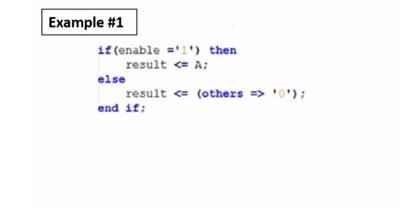In first example we have if enable =1 then result equals to A else our results equal to others 0. We have if enable =1 a conditional statement and if its verified results equal to A otherwise our result will be 0. We get to know that both A and 0 should be of same data type because the result is being in the else clause displayed as 0, if it displays as A, A should be a standard logic vector, signed or unsigned data type. So, this is a valid if statement.Let’s have a look to another example. We have if, enable + check then result is equal to A, end if. This is an if statement which is valid however our conditional statement is not equal to true or false. We just have enable + check that is not equal to 0 or 1, true or false, that can be any value. So, this is an invalid if statement. The reason behind this that conditional statement is not true or false.

VHDL Programming example 3

Let’s have a look to another example. If enable is equal to 0 then result is equal to A and end if. So, here we do not have the else clause. We just have if and end if. So, this is a valid if statement.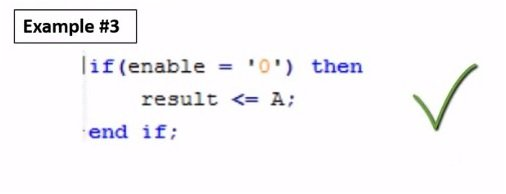### VHDL programming Multiple if else statements

With if statement, you can do multiple else if. There is no limit. VHDL supports multiple else if statements.  If, else if, else if, else if and then else and end if.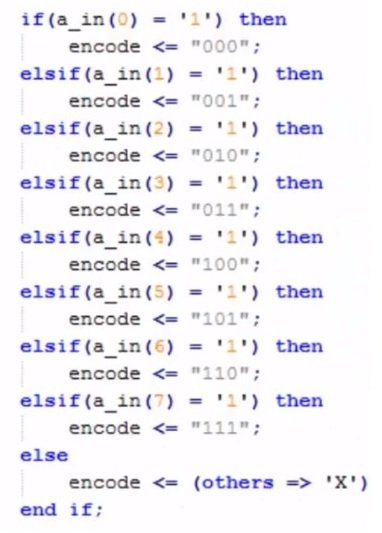Let’s take an example, is we have if a_in (0) vector equals to 1, then encode equals to 000. See for all else if, we have different values. For another a_in(1) equals to 1 we have encode equals to 001. All the way down to a_in(7) equals to 1 then encode equals to 111. Then we have else, is all of the if and else if statement are not true then we are going to in else statement. If none is true then our code is going to have an output x or undefined in VHDL language. Then we have an end if in VHDL language. You will think elseif statement is spelled as else space if but that’s not the case. It is spelled as else if. That’s certainly confusing. But if you write else space if, then it will give error, it’s an invalid syntax.

### VHDL Programming Case Statement

So let’s talk about the case statement in VHDL programming. A case statement checks input against multiple ‘cases’. The keywords for case statement are case, when and end case.

Note: when we have a case statement, it’s important to know about the direction of => and <=.

When you are working on a case statement, every option that is possible must be covered or it may make use of others keyword. So, conditions cannot overlap, if I have a case equals between 1 and 3, so in my next case if I have 2, then that’s not valid because now they overlap. My first case between 1 and 3, if my value is true my 1 and 3 is evaluated true and my 2 is also true. So, you should avoid overlapping in case statement otherwise it will give error.

For example, if we have a case, which taking value in inputs which says that if our value in input is 000 then our output is going to be 00. If we go on following the queue, same type of situation is going on. When our input is going to be 001, out output will be 01 and if we go through all set of different conditions from 000 to 111, we have different outputs. We also have others which is very good. Whenever, you have case statement, we recommend you to have others statement. The benefit of others statement is that if you forget to write any case that could have happened, then make sure you give this time of error caption. It acts as a function of safety. In this case, if all cases are not true, we have an x or an undefined case. So, conversely if you see x or undefined, you come to know that something wrong is going on in your statement or there is any kind of error.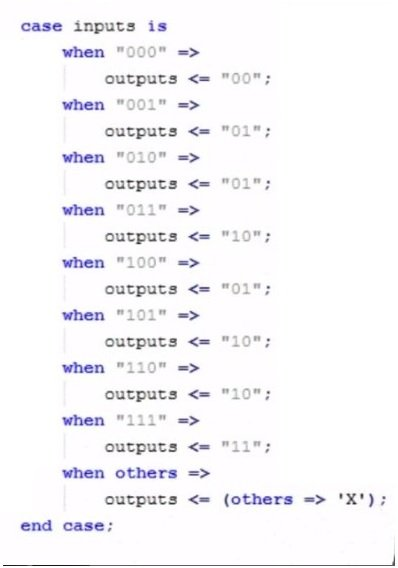### VHDL Programming If Statements Vs Case Statements

Let’s have a comparison of if statements and case statements of VHDL programming. If you look at if statement and case statement you think somehow they are similar. We have a function, we can implement same thing in if statement and in case statement. However, the major difference between the two is that If Statement infers priority, this is because if the first statement is true it will evaluate an expression and then ignore the rest of the else if. Whereas, in case statement we have to over ever possible ‘case’. So, there is as such no priority in case statement. You cannot have a situation that is overlapping whereas in if and else if statements, you may have different overlapping conditions. In case statement, every single case have same exact priority. In if statement you do not have to cover every possible case unlike case statement.

### VHDL Programming For Loop and While Loop

In this part of the article, we will describe how for loop and while loop can be used in VHDL.

For Loop

First of all, we will explain for loop. A for loop is used to generate multiple instances of same logic. In VHDL, for loops are able to go away after synthesis. We usually use for loop for the construction of the circuits. But after synthesis I goes away and helps in creating a number of codes.

The basic keywords in a for loop include

• For
• In
• To
• Loop
• .End Loop

For Loop Example

Here we have an example of for loop.

We have three signals. Signal A, B and C and a standard logic vector from 4 downto 0, 5 bits wide. And now, we have a for loop statement where we use generic or in gates. We have for in 0 to 4 loop. What we are going to do is, we are going to take which is going to be related to value from 0 to 4. So, we get five relations, 0, 1, 2, 3 and 4 and inside the value loop whatever statement we are going to play its going to be related five times. We have statement C(i) is equal to A(i) and B(i). These are generic 5 different in gates. Signals A and B will be ended together and as a result they will create signal C. In figure see we have 5 different in gates, if A(0) and B(0) then output is C(0) and the same goes on with A(1), B(1) down to A(4), B(4) with output C(4). So, it’s an easy way instead of writing C(i) equals to A(i), B(i) or C(1) equals to  A(1), B(1). This is quicker way of doing this. Here we have 5 in gates. But if you have more complex circuit where you are working say for instance 100 in gates, this is the faster way. You can also worked on more complex form, but this is a general idea. In this 4 loops example, 4 loops are going to generate 4 in gates.While Loop

Now, we will talk about while loop. In nature, it is very similar to for loop. However, there are several differences between the two.

In while loop, the condition is first checked before the loop is entered. In for loop we specifically tell a loop how many times we want to evaluate. For example, we want from 0 to 4, we will be evaluating 5 times. However, in a while loop, we have a condition and this condition I checked before we go onto the loop and every time we evaluate the loop we check that condition. If that condition evaluates as true, we get out of the loop. So, if the loop continues running, the condition evaluates as true or false. It’s important to know, the condition eventually evaluates as true or false. Let’s have a look to the syntax of while loop, how it works.

We have the loop name, while condition and this condition be whatever we want, if it’s true it’s going to execute loop statement in our loop and then after executing our statement we end our loop. So, you could do same exactly in a while loop versus a for loop, However, you have to make sure at some important times whether your condition will evaluate as true or false.

Here we have an example of while loop. For instance, we have a process which is P2, we are going to evaluate it as ln_z. A variable z1, we are going to give a value 1. Then, we begin. After that we have a while loop. While z1 is equal to less than  or equal to 99. So, our out_z is being said to ln_z(z1+8) and an important thing to note here is, z1 = Z1 + 1. Z1 starts with 1 and it goes through 99 times while z1 is less than or equal to 99. Once we are done 100 times, we get out of the loop and end our process. Now, if we take out the statement, z1 = z1 + 1, we create a condition called an infinite loop. If our while loop is never going to be false, then your loop will spin forever and this can be a problem either your synthesizer will catch this or will cause an error or your code will not process in VHDL. So, that can cause some issues. So, we actually have to be careful when we are working on a while loop.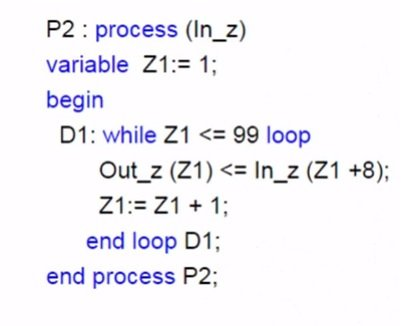Comparison of for loop and while loop

Here we have main difference between for loop and a while loop.

• For loops will iterate a specified number of times.
• While Loops will iterate until the condition becomes false.
• When you are working with a while loop, you must be very cautious of infinite loop.

### VHDL Programming  For Loop Example

While working with VHDL, many people think that we are doing programming but actually we are not. Here is a project opened in Microsoft visual studio is a C++ and work essentially going on is a for loop and i.e. we have an integer i and we are looping through it 5 times and we are outputting the value as the variable i.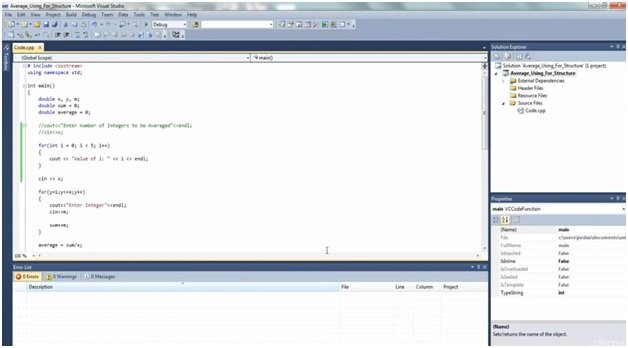Then we click on the debug option from top bar and it shows us that value of i changes from 0, 1, 2, 3 and 4.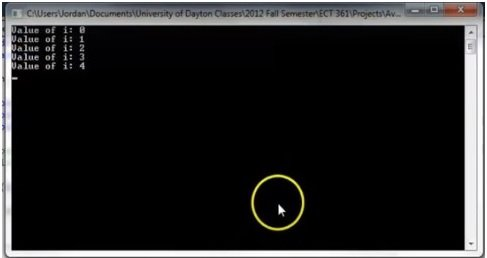Here you can see what a for loop in VHDL looks like and in the syntax section we have covered what a for loop in VHDL needs to work, file and everything like that.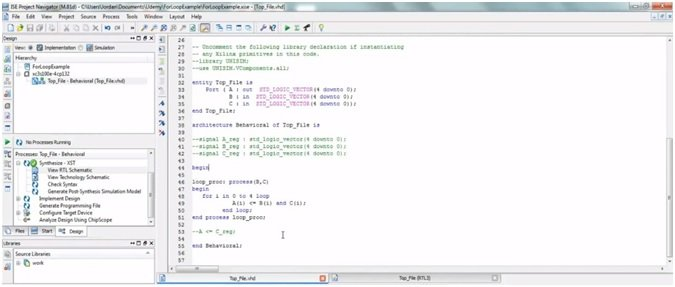Look at the line 48 and 49, we have a for loop and a variable i and we are looping from 0 to 4 which is same as we had in C++ for loop we looked at. We are taking variable A which is equal to B and C.If you are going to synthesize it, we are going to show you how the real time logic numeric. So, it’s showing how it generates. If you run this, you click on Top File RTL.We have Top File 1 which is a VHDL file and essentially and gates which are these logic vectors. 2 inputs will give us 1 output. So, this is the difference between VHDL and software.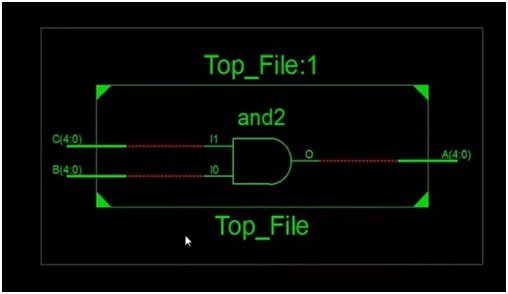In software, you are modifying value of variables whereas in hardware or in VHDL you’re describing the actual hardware. So, let’s have a look to VHDL hardware. We have a digital logic circuit, we are going to generate in VHDL.  On the left we have the inputs A, B and C. We are going to or A and B and the value of that and input C invert value in output D.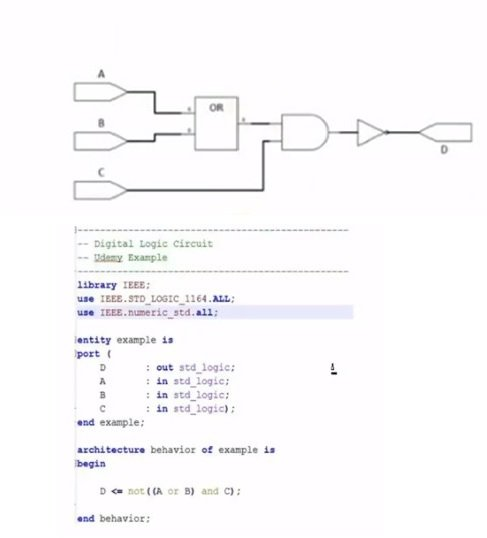So, whatever we are doing in VHDL, we are describing it in hardware work.

### VHDL Programming When-Else statement/ with-select- when statement

Here we will discuss, when select, with select and with select when statement in VHDL language.

When-Else Statement

First of all, let’s talk about when-else statement.

• A when-else statement allows a signal to be assigned a value based on set of conditions.
• This statement is considered a concurrent signal assignment, this is directly placed under the category of architecture.
• It is very similar to a case statement, except of the fact that case statement can only be placed in VHDL process whereas a when-else statement don’t need to be placed in the process.

### VHDL Programming Example of When-Else Statement

Here we have an example of when-else statement. We have two signals a and b. the standard logic vector of signal b is from 3 down to 0 so its 4 bits wide and of signal a is 1 down to 0 so its 2 bits wide. Then we use our when-else statement. Our when-else statement is going to assign value to b depending upon the value of a. In first line, see value of b is 1000 when a is equal to 00 otherwise b will be equal to 0100 when a is equal to 01. B equal to 0010 when a equal to 10 and b equal to 0001 when a equal to 11.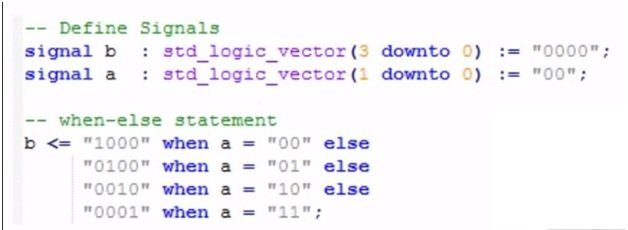Now, if you look at this statement, you can say that I can implement it in case statement. In VHDL as well as other languages, you can do a lot of same things by choosing different coding styles, different statements or structures. So, in this case you want something to put directly into the architecture and you want it to happen before clk edge, you will use a when-else statement. However, if you need to rise it or fall it or evaluate a signal every time a signal changes state, you will use a case statement and place it in process instead of architecture.

With-select-when Statement

This allows one of several possible values to be assigned to a signal based on select expression.

The choices selected must be determinable when you are going to compile them.

Somehow, this has similarities with case statement. So, with-select statement and with-select-when statement are very similar to same exact things and are in preference to be used.

Example of with-select-when statement

We are working with a with-select-when statement. We have an example. We have with a select, y is equal to c0 when 000 or to c1 when 001, c2 when 010 and c3 when 011. Then, we have 0 when others.

VHDL Processes and Concurrent Statement

In this part of article, we are going to talk about the processes in VHDL and concurrent statements.

### VHDL Programming Processes

In VHDL Process a value is said to determine how we want to evaluate our signal. The signal is evaluated when a signal changes its state in sensitivity. So, any signal we put in sensitivity of a process. If it goes from high to low, if you have a standard logic vector in it and that goes from high to low that process is evaluated.

There are several parts in VHDL process that include

• Name
• Sensitivity List
• Begin Statement
• End process Statement

The name is what we use to name the process. If we have multiple process in our design, the name is used to organize the structure, if you talk to someone you can define the process. The sensitivity list is used to determine when our process will be evaluated. The begin statement tells us where our process actually starts. So, we have our process and we can change our variables and then we tell to begin and then we have our end process statement.

Examples of VHDL Process

It’s very interesting to look at VHDL Process example. We have a name which is stated as state_process then we give semi colon and write process and sensitivity list. In the sensitivity list, we have a clk which is common signal input in our process but the clk starts going from low to high or high to low, every time it makes a transition, this process get evaluated. When it goes high, process is evaluated and when it gets lower, the process is again evaluated. Then we have ‘begin’ i.e. we actually start our evaluation process and inside process we have simple if else statement. If it’s a rising_edge our clk then we check the second statement if reset is equals to 0 then we have stated is equal to init else our state value is equal to nxt_state. So, every time when our clk is at rising edge, we will evaluate the if else and if statement. You don’t have to put a clk because the standard logic vector integer or any signal inside the process determine when you want to evaluate that process.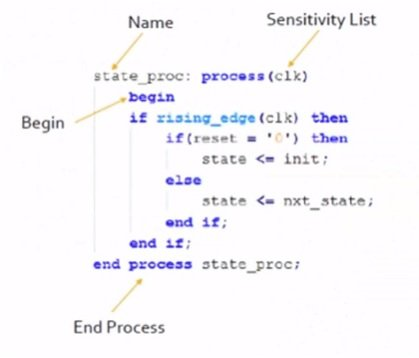### VHDL Programming Concurrent Signal Assignments

Here we will discuss concurrent signal assignments.

The concurrent signal assignments are used to assign a specific value to a signal inside your VHDL design. Signals can be assigned as certain vales such as 1 or 0 or you can have an integer value that you set 1, 2, 3, 4, 5, 6 so on and so far. The big thing to know about signal assignment is that these are concurrent so so if the top of the design we have A equals to 1 and C equals to 0. A is said to 1 and at the same time C is said to 0. These things happen concurrently, there is no order that this happens first and then this happens second. They happen in same exact time. Let’s look how we do concurrent signal assignments. We have next state of certain value of state. So, state and next state have to be of the same data type. We cannot assign two different data types. They have to be the same data types. Signal assignments are always happening. These are not sequential operations.

Example of Concurrent Signal Assignments

The important thing to know is that at the exact same time, next state is getting the value of state and data ready is getting the value of 0. So, we can rearrange this order and the outputs are going to be same. Our design is going to act as same. All this happens simultaneously. There is no order, one happens first then next happens so and so far. We have advantage of this parallelism while working on FPGA and VHDL. We can say this happens and at the same exact time the other happens.VHDL Coding Structure

Here we have VHDL Syntax design example.Here is Universal Shift Register VHDL File and we want to show you adjacent uses of different keywords. Starting with line 1, we have a comment which is USR, it’s going to be header. Then we have library which is highlighted in blue and IEEE in red. Then we have use IEEE standard logic vector and signed or unsigned data type. Then moving forward, we have entity, generic, data width is a type of an integer. Our A is a standard logic vector. I on line 11 is also a standard logic vector. S is again standard logic vector whereas reset and clk are standard logic values. In line 17, we have architecture. We have signal which we call A_reg on line 19 which is a standard logic vector and data width -1 downt 0.

If we give data width 8 to A then 8-1 equals to 7 downto 0. So, it gives us A-reg 8 bits wide because 7 downto 0 gives us 8 different values. Look at line 21, we have ‘begin’ keyword, at line 27 we got if rising edge as a keyword as well which indicates that when our clk when changes its state, if it is at rising edge then the value is true whereas on falling edge it is not true. At line 31 we have a case statement. When 00, we are taking in our case S which is an input in standard logic vector, 2 downto 0 which gives us value 3. Then, you can see there are different values given to S i.e. When 00 hold, when 01 right shift, when 10 left shift, when 11 parallel load. We also have ‘when others’ which is an error code which gives us that we have register of a value of an x which is just like an undetermined value. The value of X means undefined, uninitialized or there is some kind of error. It’s a test for you. After that you can check your coding structure. It makes easier to grab your error.

So this is all about VHDL programming tutorial and coding guide. In next articles, I will write about more examples with VHDL programming. If you like this tutorial, please don’t forget to share it with your friends also.

### 2 thoughts on “VHDL programming if else statements and loops with examples”

1. NICE EXPLANATION, WE UNDERSTOOD VERY WELL. THANKS FOR INFORMATION.